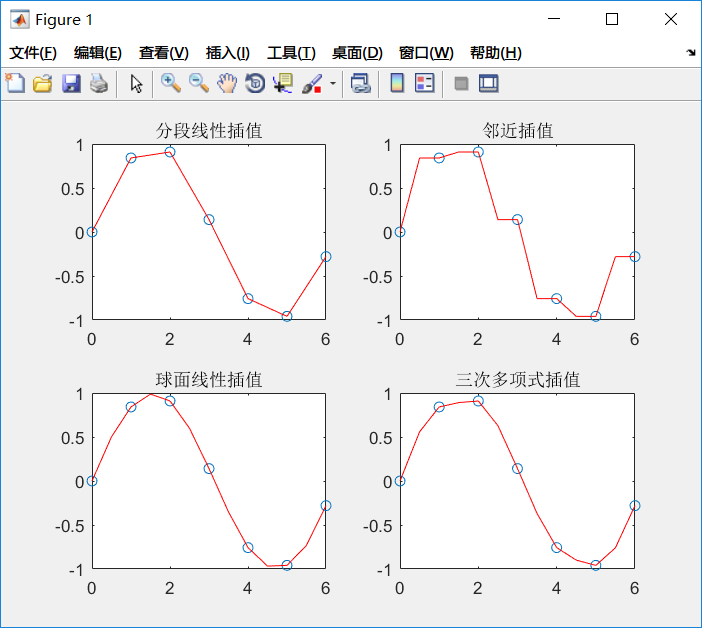# Matlab—回归与内插（完结）

## November 22, 2018 • Read: 701 • matlab

### 拟合（回归）与内插

#### polyfit()

x = [-1.2 -0.5 0.3 0.9 1.0 2.6 3.0 3.5];
y = [-15.6 -8.5 2.2 4.5 6.6 8.2 8.9 10.0];
fit = polyfit(x,y,7);
xfit = x(1):0.1:x(end);
yfit = polyval(fit,xfit);
plot(x,y,'ro',xfit,yfit);#### scatter() & corrcoef()

x = [-1.2 -0.5 0.3 0.9 1.0 2.6 3.0 3.5];
y = [-15.6 -8.5 2.2 4.5 6.6 8.2 8.9 10.0];
scatter(x,y);
corrcoef(x,y)#### regress()

Regress函数的作用也是做数据拟合，只不过它所呈现的信息更多，其调用格式为:[b,bint,r,rint,stats] = regress(y,x,alpha)

• y：多元拟合的变量值的向量
• x 多元拟合的自变量的值的矩阵
• alpha：显著性水平，缺省的时候为 0.05
• b：回归得到的自变量系数
• bint：b 的 95% 的置信区间矩阵
• r：残差向量
• rint：区间矩阵

#### 插值

y=interp1(x,Y,xi)由已知点集 (x,Y) 插值计算 xi 上的函数值
y=interp1(x,Y,xi)相当于 x=1:length(Y) 的 interp(x,Y,xi)
y=interp1(x,Y,xi,method)

y=interp1(x,Y,xi,method,'extrap') | 对 xi 中超出已知点集的插值点用指定插值方法计算函数值
y=interp1(x,Y,xi,method,'extrap',extrapval) | 用指定方法插值 xi 上的函数值，超出已知点集处函数值取 extrapval
y=interp1(x,Y,xi,method,'pp') |

Method方法描述
Nearest最邻近插值: 插值点处函数值与插值点最邻近的已知点函数值相等
liner分段线性插值：插值点处函数值由连接其最邻近的两侧点的线性函数预测。Matlab 中 interp1 的默认方法
spline样条插值：默认为三次样条插值。可用 spline 函数替代
pchip三次 Hermite 多项式插值，可用 pchip 函数替代
1. Nearest方法速度最快，占用内存最小，但一般来说误差最大，插值结果最不光滑
2. Spline三次样条插值是所有插值方法中运行耗时最长的，插值函数及其一二阶导函数都连续，是最光滑的插值方法。占用内存比 cubic 方法小，但是已知数据分布不均匀的时候可能出现异常结果
3.  Cubic三次多项式插值法中，插值函数及其一阶导数都是连续的，所以插值结果比较光滑，速度比 Spline 快，但是占用内存最多
x = 0 : 2 * pi;
y = sin(x);
xx = 0 : 0.5 : 2 * pi;
%interp1对sin函数进行分段线性插值，调用interp1的时候，默认的是分段线性插值
y1 = interp1(x,y,xx);
subplot(2,2,1);plot(x,y,'o',xx,y1,'r');
title('分段线性插值')

%临近插值
y2 = interp1(x,y,xx,'nearnest');
subplot(2,2,2);plot(x,y,'o',xx,y2,'r');
title('邻近插值')

%球面线性插值
y3 = interp1(x,y,xx,'spline');
subplot(2,2,3);plot(x,y,'o',xx,y3,'r');
title('球面线性插值')

%三次多项式插值
y4 = interp1(x,y,xx,'PCHIP');
subplot(2,2,4);plot(x,y,'o',xx,y4,'r');
title('三次多项式插值')### 结语

Matlab系列文章已全部更完，如有任何错误或者建议欢迎指出，同时欢迎各位读者加入博客交流群 689696631。最后，如果你是看了我的系列文章准备参加美赛，祝你取得好成绩